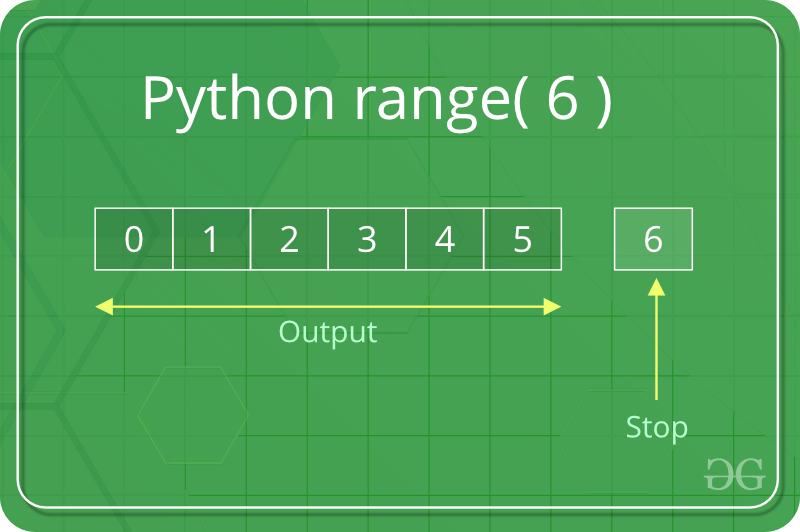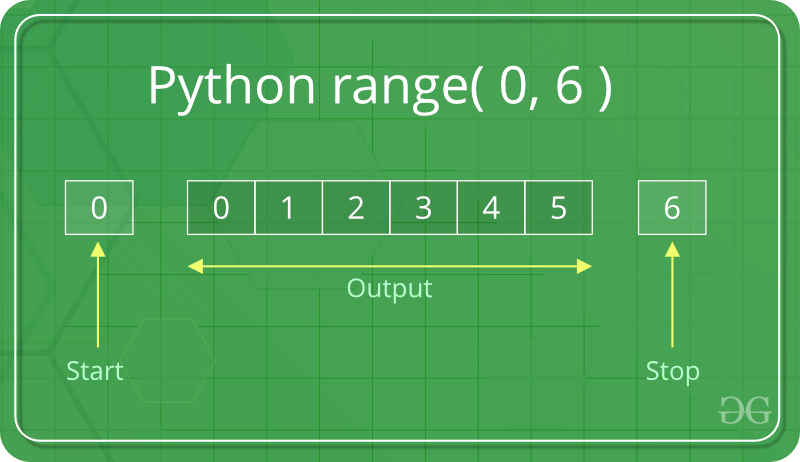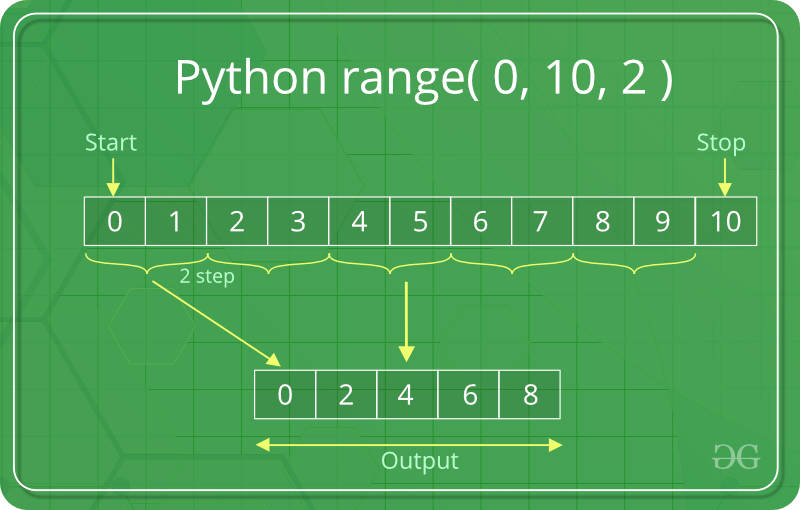Related Articles

# Python range() function

• Difficulty Level : Easy
• Last Updated : 23 Sep, 2021

Python range() function returns the sequence of the given number between the given range.

range() is a built-in function of Python. It is used when a user needs to perform an action a specific number of times. range() in Python(3.x) is just a renamed version of a function called xrange in Python(2.x). The range() function is used to generate a sequence of numbers.

Python range() function for loop is commonly used hence, knowledge of same is the key aspect when dealing with any kind of Python code. The most common use of range() function in Python is to iterate sequence type (Python range() List, string, etc. ) with for and while loop.

### Python range syntax

range(stop)

range(start, stop[, step])

### Python range() Basics

In simple terms, range() allows the user to generate a series of numbers within a given range. Depending on how many arguments the user is passing to the function, user can decide where that series of numbers will begin and end as well as how big the difference will be between one number and the next.range() takes mainly three arguments.

• start: integer starting from which the sequence of integers is to be returned
• stop: integer before which the sequence of integers is to be returned. The range of integers end at stop – 1.
• step: integer value which determines the increment between each integer in the sequence

## Python3

 `# Python Program to``# show range() basics` `# printing a number``for` `i ``in` `range``(``10``):``    ``print``(i, end``=``" "``)``print``()` `# using range for iteration``l ``=` `[``10``, ``20``, ``30``, ``40``]``for` `i ``in` `range``(``len``(l)):``    ``print``(l[i], end``=``" "``)``print``()` `# performing sum of natural``# number``sum` `=` `0``for` `i ``in` `range``(``1``, ``11``):``    ``sum` `=` `sum` `+` `i``print``(``"Sum of first 10 natural number :"``, ``sum``)`

Output :

```0 1 2 3 4 5 6 7 8 9
10 20 30 40
Sum of first 10 natural number : 55```

### There are three ways you can call range() :

• range(stop) takes one argument.
• range(start, stop) takes two arguments.
• range(start, stop, step) takes three arguments.

## range(stop)

When user call range() with one argument, user will get a series of numbers that starts at 0 and includes every whole number up to, but not including, the number that user have provided as the stop. For Example –## Python3

 `# Python program to``# print whole number``# using range()` `# printing first 10``# whole number``for` `i ``in` `range``(``10``):``    ``print``(i, end``=``" "``)``print``()` `# printing first 20``# whole number``for` `i ``in` `range``(``20``):``    ``print``(i, end``=``" "``)`

Output:

```0 1 2 3 4 5 6 7 8 9
0 1 2 3 4 5 6 7 8 9 10 11 12 13 14 15 16 17 18 19 ```

## range(start, stop)

When user call range() with two arguments, user get to decide not only where the series of numbers stops but also where it starts, so user don’t have to start at 0 all the time. User can use range() to generate a series of numbers from X to Y using a range(X, Y). For Example -arguments## Python3

 `# Python program to``# print natural number``# using range` `# printing a natural``# number upto 20``for` `i ``in` `range``(``1``, ``20``):``    ``print``(i, end``=``" "``)``print``()` `# printing a natural``# number from 5 t0 20``for` `i ``in` `range``(``5``, ``20``):``    ``print``(i, end``=``" "``)`

Output:

```1 2 3 4 5 6 7 8 9 10 11 12 13 14 15 16 17 18 19
5 6 7 8 9 10 11 12 13 14 15 16 17 18 19 ```

## range(start, stop, step)

When the user call range() with three arguments, the user can choose not only where the series of numbers will start and stop but also how big the difference will be between one number and the next. If the user doesn’t provide a step, then range() will automatically behave as if the step is 1.

## Python3

 `# Python program to``# print all number``# divisible by 3 and 5` `# using range to print number``# divisible by 3``for` `i ``in` `range``(``0``, ``30``, ``3``):``    ``print``(i, end``=``" "``)``print``()` `# using range to print number``# divisible by 5``for` `i ``in` `range``(``0``, ``50``, ``5``):``    ``print``(i, end``=``" "``)`

Output :

```0 3 6 9 12 15 18 21 24 27
0 5 10 15 20 25 30 35 40 45 ```In this example, we are printing an even number between 0 to 10 so we choose our starting point from 0(start = 0) and stop the series at 10(stop = 10). For printing even number the difference between one number and the next must be 2 (step = 2) after providing a step we get a following output ( 0, 2, 4, 8).

### Example 5: Incrementing with the range using positive step

If a user wants to increment, then the user needs steps to be a positive number. For example:

## Python3

 `# Python program to``# increment with``# range()` `# incremented by 2``for` `i ``in` `range``(``2``, ``25``, ``2``):``    ``print``(i, end``=``" "``)``print``()` `# incremented by 4``for` `i ``in` `range``(``0``, ``30``, ``4``):``    ``print``(i, end``=``" "``)``print``()` `# incremented by 3``for` `i ``in` `range``(``15``, ``25``, ``3``):``    ``print``(i, end``=``" "``)`

Output :

```2 4 6 8 10 12 14 16 18 20 22 24
0 4 8 12 16 20 24 28
15 18 21 24 ```

### Example 6: Python range() backwords

If a user wants to decrement, then the user needs steps to be a negative number. For example:

## Python3

 `# Python program to``# decrement with``# range()` `# incremented by -2``for` `i ``in` `range``(``25``, ``2``, ``-``2``):``    ``print``(i, end``=``" "``)``print``()` `# incremented by -4``for` `i ``in` `range``(``30``, ``1``, ``-``4``):``    ``print``(i, end``=``" "``)``print``()` `# incremented by -3``for` `i ``in` `range``(``25``, ``-``6``, ``-``3``):``    ``print``(i, end``=``" "``)`

Output :

```25 23 21 19 17 15 13 11 9 7 5 3
30 26 22 18 14 10 6 2
25 22 19 16 13 10 7 4 1 -2 -5 ```

### Example 7: Python range() float

Python range() function doesn’t support the float numbers. i.e. user cannot use floating-point or non-integer number in any of its argument. Users can use only integer numbers. For example

## Python3

 `# Python program to``# show using float``# number in range()` `# using a float number``for` `i ``in` `range``(``3.3``):``    ``print``(i)` `# using a float number``for` `i ``in` `range``(``5.5``):``    ``print``(i)`

Output :

```for i in range(3.3):
TypeError: 'float' object cannot be interpreted as an integer```

### Example 8: Concatenation of two range() functions

The result from two range() functions can be concatenated by using the chain() method of itertools module. The chain() method is used to print all the values in iterable targets one after another mentioned in its arguments.

## Python3

 `# Python program to concatenate``# the result of two range functions``from` `itertools ``import` `chain` `# Using chain method``print``(``"Concatenating the result"``)``res ``=` `chain(``range``(``5``), ``range``(``10``, ``20``, ``2``))` `for` `i ``in` `res:``    ``print``(i, end``=``" "``)`

Output:

```Concatenating the result
0 1 2 3 4 10 12 14 16 18 ```

### Example 9: Accessing range() with index value

A sequence of numbers is returned by the range() function as its object that can be accessed by its index value. Both positive and negative indexing is supported by its object.

## Python3

 `# Python program to demonstrate``# range function` `ele ``=` `range``(``10``)[``0``]``print``(``"First element:"``, ele)` `ele ``=` `range``(``10``)[``-``1``]``print``(``"\nLast element:"``, ele)` `ele ``=` `range``(``10``)[``4``]``print``(``"\nFifth element:"``, ele)`

Output:

```First element: 0

Last element: 9

Fifth element: 4```

Points to remember about Python range() function :

• range() function only works with the integers i.e. whole numbers.
• All arguments must be integers. Users can not pass a string or float number or any other type in a start, stop and step argument of a range().
• All three arguments can be positive or negative.
• The step value must not be zero. If a step is zero python raises a ValueError exception.
• range() is a type in Python
• Users can access items in a range() by index, just as users do with a list:

Attention geek! Strengthen your foundations with the Python Programming Foundation Course and learn the basics.

To begin with, your interview preparations Enhance your Data Structures concepts with the Python DS Course. And to begin with your Machine Learning Journey, join the Machine Learning – Basic Level Course

My Personal Notes arrow_drop_up